Download Presentation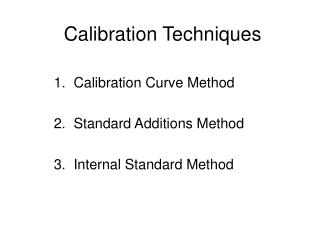Calibration Techniques

# Calibration Techniques - PowerPoint PPT Presentation

Calibration Techniques. 1. Calibration Curve Method 2. Standard Additions Method 3. Internal Standard Method. Calibration Curve Method. Most convenient when a large number of similar samples are to be analyzed. Most common technique. Facilitates calculation of Figures of Merit.I am the owner, or an agent authorized to act on behalf of the owner, of the copyrighted work described.
Download Presentation## Calibration Techniques

An Image/Link below is provided (as is) to download presentation

Download Policy: Content on the Website is provided to you AS IS for your information and personal use and may not be sold / licensed / shared on other websites without getting consent from its author.While downloading, if for some reason you are not able to download a presentation, the publisher may have deleted the file from their server.

- - - - - - - - - - - - - - - - - - - - - - - - - - E N D - - - - - - - - - - - - - - - - - - - - - - - - - -
Presentation Transcript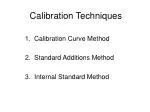### Calibration Techniques

1. Calibration Curve Method

2. Standard Additions Method

3. Internal Standard Method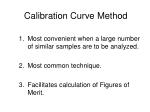### Calibration Curve Method

Most convenient when a large number of similar samples are to be analyzed.

Most common technique.

Facilitates calculation of Figures of Merit.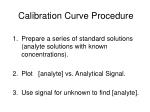### Calibration Curve Procedure

Prepare a series of standard solutions (analyte solutions with known concentrations).

Plot [analyte] vs. Analytical Signal.

Use signal for unknown to find [analyte].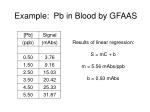### Example: Pb in Blood by GFAAS

Results of linear regression:

S = mC + b

m = 5.56 mAbs/ppb

b = 0.93 mAbs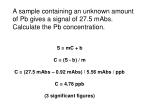A sample containing an unknown amount of Pb gives a signal of 27.5 mAbs. Calculate the Pb concentration.

S = mC + b

C = (S - b) / m

C = (27.5 mAbs – 0.92 mAbs) / 5.56 mAbs / ppb

C = 4.78 ppb

(3 significant figures)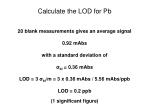Calculate the LOD for Pb

20 blank measurements gives an average signal

0.92 mAbs

with a standard deviation of

σbl = 0.36 mAbs

LOD = 3 σbl/m = 3 x 0.36 mAbs / 5.56 mAbs/ppb

LOD = 0.2 ppb

(1 significant figure)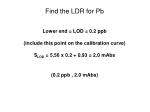Find the LDR for Pb

Lower end = LOD = 0.2 ppb

(include this point on the calibration curve)

SLOD = 5.56 x 0.2 + 0.93 = 2.0 mAbs

(0.2 ppb , 2.0 mAbs)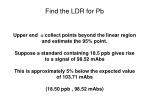Find the LDR for Pb

Upper end = collect points beyond the linear region and estimate the 95% point.

Suppose a standard containing 18.5 ppb gives rise to s signal of 98.52 mAbs

This is approximately 5% below the expected value of 103.71 mAbs

(18.50 ppb , 98.52 mAbs)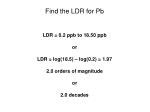Find the LDR for Pb

LDR = 0.2 ppb to 18.50 ppb

or

LDR = log(18.5) – log(0.2) = 1.97

2.0 orders of magnitude

orFind the Linearity

Calculate the slope of the log-log plotRemember

S = mC + b

log(S) = log (mC + b)

b must be ZERO!!

log(S) = log(m) + log(C)

The original curve did not pass through the origin. We must subtract the blank signal from each point.### Standard Addition Method

Most convenient when a small number of samples are to be analyzed.

Useful when the analyte is present in a complicated matrix and no ideal blank is available.### Standard Addition Procedure

Add one or more increments of a standard solution to sample aliquots of the same size. Each mixture is then diluted to the same volume.

Prepare a plot of Analytical Signal versus:

volume of standard solution added, or

concentration of analyte added.### Standard Addition Procedure

The x-intercept of the standard addition plot corresponds to the amount of analyte that must have been present in the sample (after accounting for dilution).

The standard addition method assumes:

the curve is linear over the concentration range

the y-intercept of a calibration curve would be 0### Example: Fe in Drinking Water

The concentration of the Fe standard solution is 11.1 ppm

All solutions are diluted to a final volume of 50 mL[Fe] = ?

x-intercept = -6.08 mL

Therefore, 10 mL of sample diluted to 50 mL would give a signal equivalent to 6.08 mL of standard diluted to 50 mL.

Vsam x [Fe]sam = Vstd x [Fe]std

10.0 mL x [Fe] = 6.08 mL x 11.1 ppm

[Fe] = 6.75 ppm### Internal Standard Method

Most convenient when variations in analytical sample size, position, or matrix limit the precision of a technique.

May correct for certain types of noise.### Internal Standard Procedure

Prepare a set of standard solutions for analyte (A) as with the calibration curve method, but add a constant amount of a second species (B) to each solution.

Prepare a plot of SA/SB versus [A].### Notes

The resulting measurement will be independent of sample size and position.

Species A & B must not produce signals that interfere with each other. Usually they are separated by wavelength or time.### Example: Pb by ICP Emission

Each Pb solution contains 100 ppm Cu.